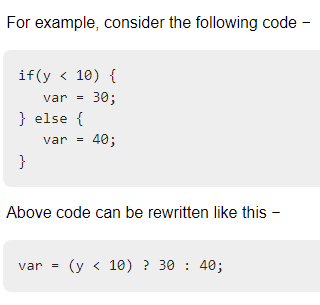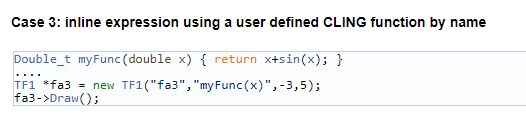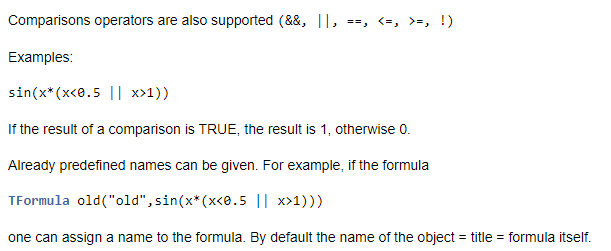# The usage of comparison and condition operators in the formula in TF1

Hi to all,

I have seen the comparison operators can be used in the equation part of TF1. Example:
TF1 * f1= new TF1(“f1”, “((x < ) *  * (x - )) + ”, 0, 100 );
I wonder if we can also use the condition operators in the equation part? Example:If it’s not possible, can “the way around it” be the inline expressions using a user defined Cling function by name as stated in TF1 link here? I also attach sample down below.I also checked the TFormula, but I came to conclusion after reading some comments that we cannot REPLACE the equation part of TF1 with TFormula. Plus, there is not much example about the usage of TFormula. Please, refer me some simple TFormula application if there is any somewhere. On the TFormula link, there is an example which I suspect the equation is missing " " quotation mark. Can you confirm that! I put the part down below.Cheers.

Are you trying to say ternary operators work fine in TF1? I see it does, I doubt one second after not seeing any example.

Is this statement correct?

TF1*total = total = new TF1(“total”, "gausn(0) + gausn(3) + ( (x< ) ? ( * (x - )) +  : ( * (x - )) +  ) ", 400 , 534);

Cheers.

``````{
TF1 *total = new TF1("total", "gausn(0) + gausn(3) + ((x < ) ?  : ) * (x - ) + ", 400., 534.);
total->SetParameters(1000., 430., 2., 2000., 500., 4., 460., 100., -4., -1.);
total->SetNpx(1000);
total->Draw();